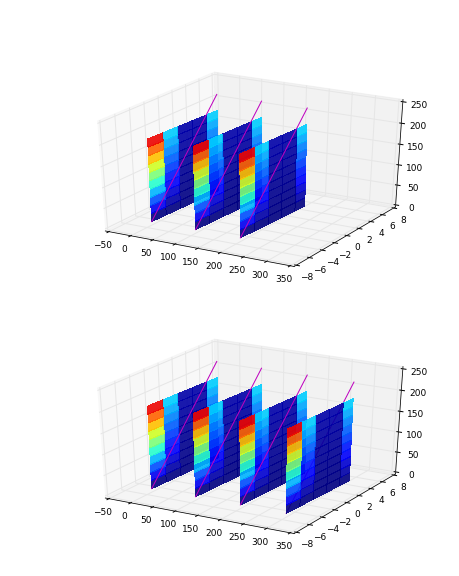# curtain plots

Hi all,

I am trying to make 3-D “curtain” plots. Basically, x,y are N-vectors, z is an M-vector, and C is MxN data set collected on the path with z. Application is a ship’s track through the ocean. I also want to be able to contour a second variable C2 also MxN. I know how to do that, but the example below just uses plot3D, because thats how I do the curtain contouring.

If I plot three such “curtains” they look OK, including the magenta line in each.

If I plot a fourth, the magenta line is obscured by the curtain, and so on for more curtains.Any clue what the problem is? The code for this example is below, and I think is self contained, plus or minus running in pylab.

Thanks, Jody

from mpl_toolkits.mplot3d import Axes3D
from matplotlib import cm
import matplotlib.pyplot as plt
import numpy as np

n=0
fig = figure()
for Nn in array([3,4]):
n=n+1
for off in arange(0,Nn*2,2)*50.:

``````    x = np.arange(-5, 5, .5)
y = np.arange(-5, 5, .5)
Z = np.arange(0,200,1)
Z=np.tile(np.reshape(Z,(200,1)),(1,size(y)))
X = np.tile(y,(200,1))
Y = np.tile(y,(200,1))

N = X*Y*Z
N = N/N.max()  # normalize 0..1
surf = ax.plot_surface(
X+off, Y, Z, rstride=20, cstride=4,
facecolors=cm.jet(N),
ax.plot(x+off+0.001,y,(y+5)*25.,'m')
ax.set_xlim([-50,350])
ax.set_ylim([-8.,8.])
``````
···

Jody Klymak
http://web.uvic.ca/~jklymak/

Hi All,

To follow up on my own post - because my curtains and contours were well-ordered, I simply set the “zorder” on each call and got the right effect.

Thanks, Jody

···

On Sep 25, 2013, at 15:15 PM, Jody Klymak <jklymak@…4192…> wrote:

Hi all,

I am trying to make 3-D “curtain” plots. Basically, x,y are N-vectors, z is an M-vector, and C is MxN data set collected on the path with z. Application is a ship’s track through the ocean. I also want to be able to contour a second variable C2 also MxN. I know how to do that, but the example below just uses plot3D, because thats how I do the curtain contouring.

If I plot three such “curtains” they look OK, including the magenta line in each.

If I plot a fourth, the magenta line is obscured by the curtain, and so on for more curtains.

Any clue what the problem is? The code for this example is below, and I think is self contained, plus or minus running in pylab.

Thanks, Jody

from mpl_toolkits.mplot3d import Axes3D
from matplotlib import cm
import matplotlib.pyplot as plt
import numpy as np

n=0
fig = figure()
for Nn in array([3,4]):
n=n+1
for off in arange(0,Nn*2,2)*50.:

``````    x = np.arange(-5, 5, .5)
y = np.arange(-5, 5, .5)
Z = np.arange(0,200,1)
Z=np.tile(np.reshape(Z,(200,1)),(1,size(y)))
X = np.tile(y,(200,1))
Y = np.tile(y,(200,1))

N = X*Y*Z
N = N/N.max()  # normalize 0..1
surf = ax.plot_surface(
X+off, Y, Z, rstride=20, cstride=4,
facecolors=cm.jet(N),
ax.plot(x+off+0.001,y,(y+5)*25.,'m')
ax.set_xlim([-50,350])
ax.set_ylim([-8.,8.])
``````

Jody Klymak
http://web.uvic.ca/~jklymak/

October Webinars: Code for Performance